# python3读取图片并灰度化图片的四种方法(OpenCV、PIL.Image、TensorFlow方法)总结

import numpy as np
import cv2
import tensorflow as tf
from PIL import Image

    img = cv2.imread(imgfile, cv2.IMREAD_GRAYSCALE)
print('大小：{}'.format(img.shape))
print("类型：%s"%type(img))
print(img)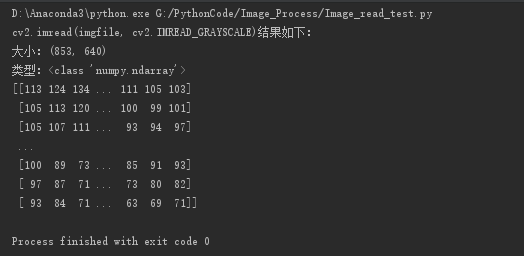img = cv2.imread(imgfile)
#print(img.shape)
#print(img)
gray_img = cv2.cvtColor(img, cv2.COLOR_BGR2GRAY) #Y = 0.299R + 0.587G + 0.114B
print("cv2.cvtColor(img, cv2.COLOR_BGR2GRAY)结果如下：")
print('大小:{}'.format(gray_img.shape))
print("类型：%s" % type(gray_img))
print(gray_img)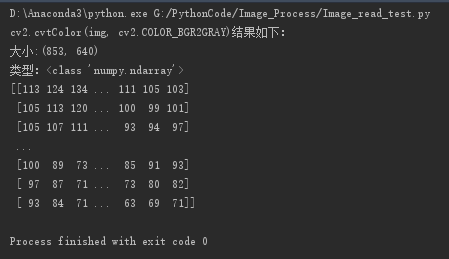img = np.array(Image.open(imgfile).convert('L'), 'f') #读取图片，灰度化，转换为数组，L = 0.299R + 0.587G + 0.114B。'f'为float类型
print("Image方法的结果如下：")
print('大小：{}'.format(img.shape))
print("类型：%s" % type(img))
print(img)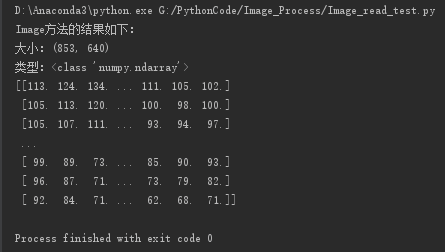with tf.Session() as sess:
img_data = tf.image.decode_jpeg(img, channels=3) #解码
#img_data = sess.run(tf.image.decode_jpeg(img, channels=3))
img_data = sess.run(tf.image.rgb_to_grayscale(img_data)) #灰度化
print('大小：{}'.format(img_data.shape))
print("类型：%s" % type(img_data))
print(img_data)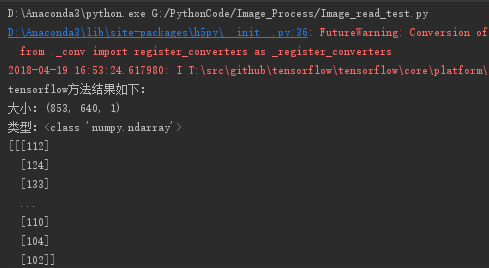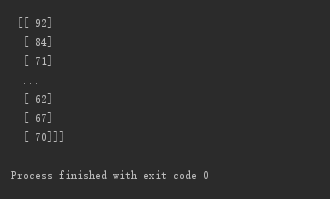12-172万+

#### Python读取图像并显示灰度图04-068万+

#### python 读取、保存、二值化、灰度化图片+opencv处理图片的方法

10-014万+

#### python中PIL.Image和OpenCV图像格式相互转换

08-153669

#### Python-Opencv 将图片转为灰度图的两种方法

09-082816

#### python骚操作——图片灰度处理

05-137996

#### python彩色图转化为灰度图

12-165006

#### OpenCV入门二：OpenCV读取一张图片显示，并将其重写为灰度图

03-251万+

#### [Python图像处理] 十四.基于OpenCV和像素处理的图像灰度化处理©️2020 CSDN 皮肤主题: 编程工作室 设计师: CSDN官方博客点击重新获取扫码支付1.余额是钱包充值的虚拟货币，按照1:1的比例进行支付金额的抵扣。
2.余额无法直接购买下载，可以购买VIP、C币套餐、付费专栏及课程。余额充值